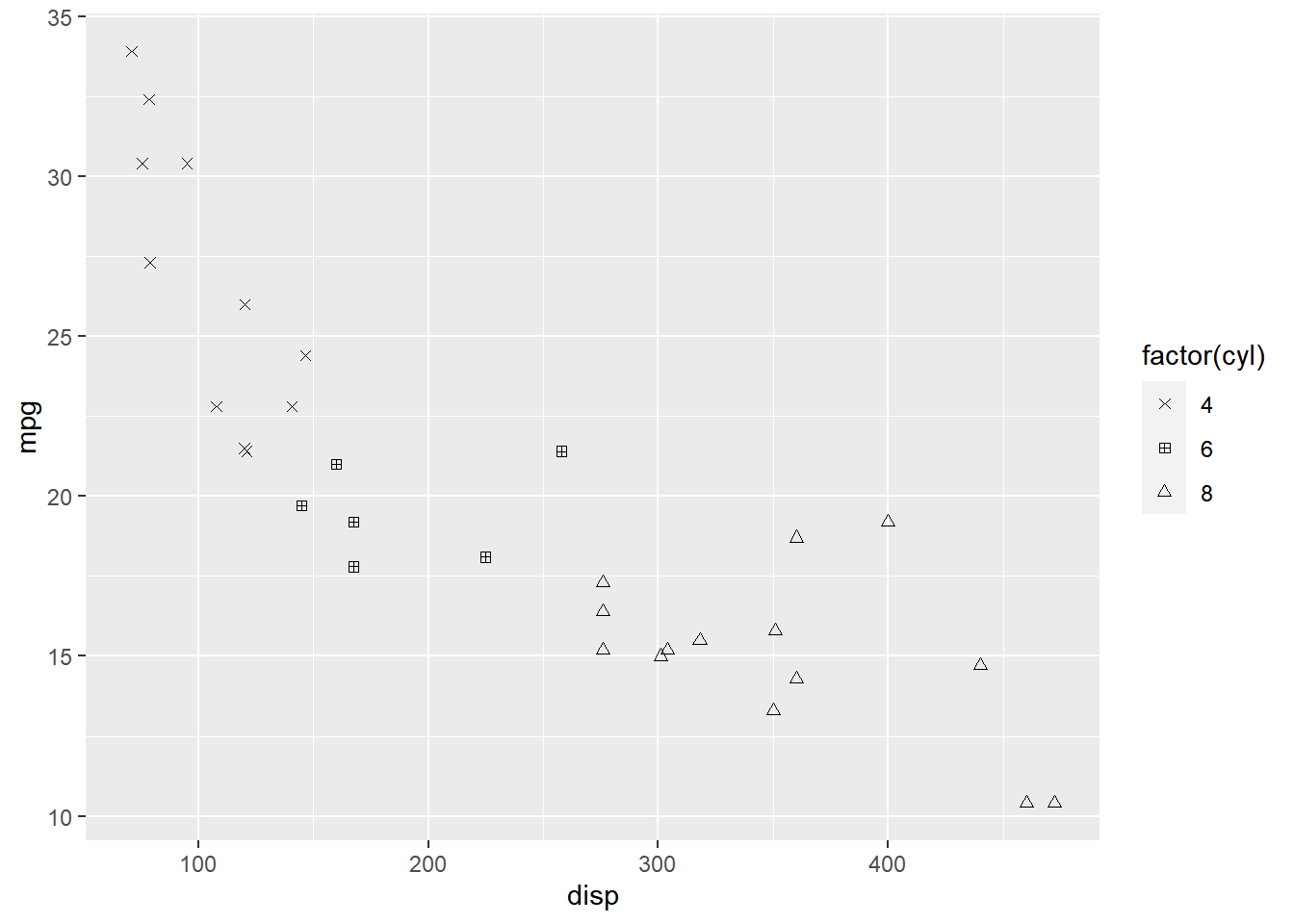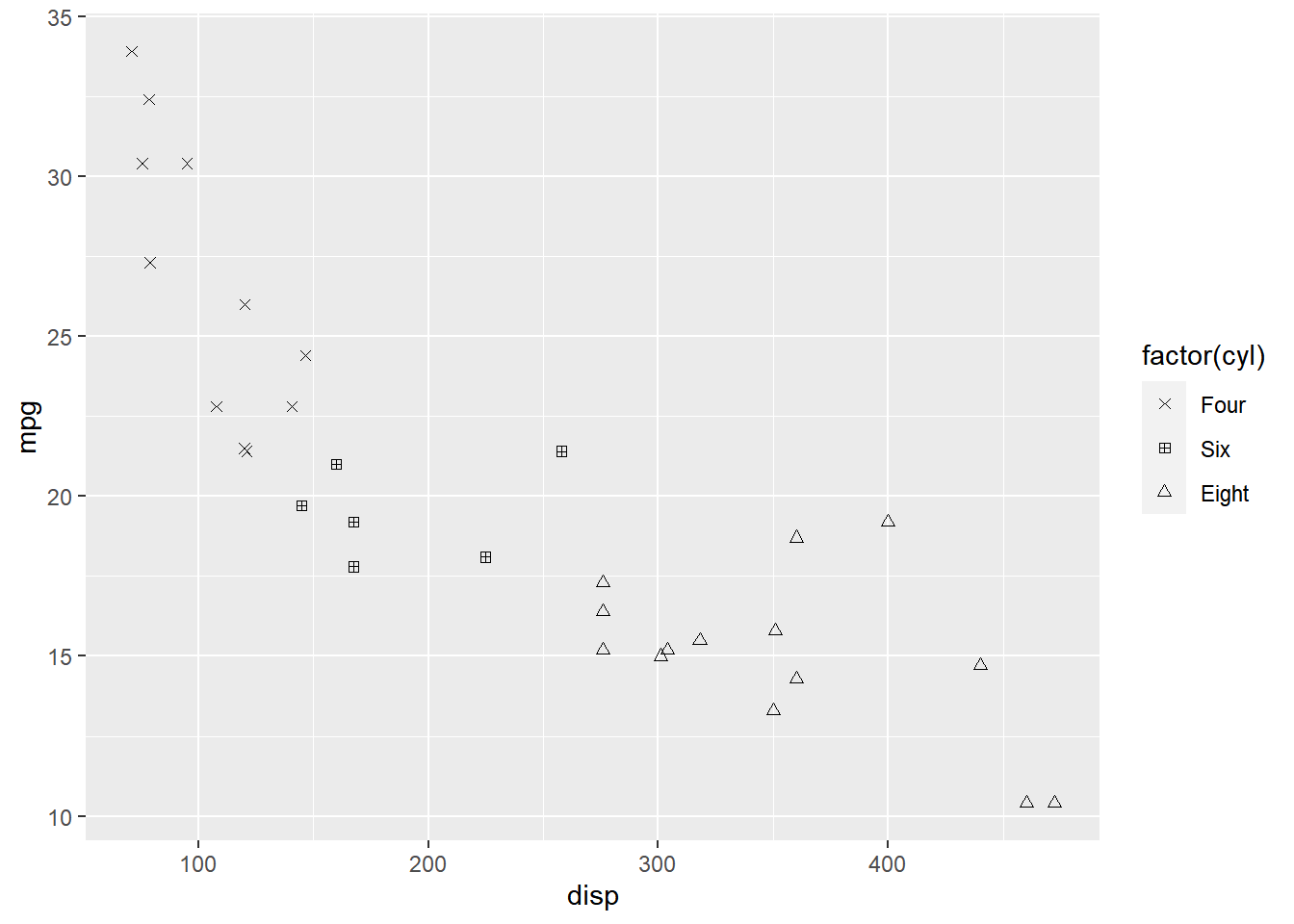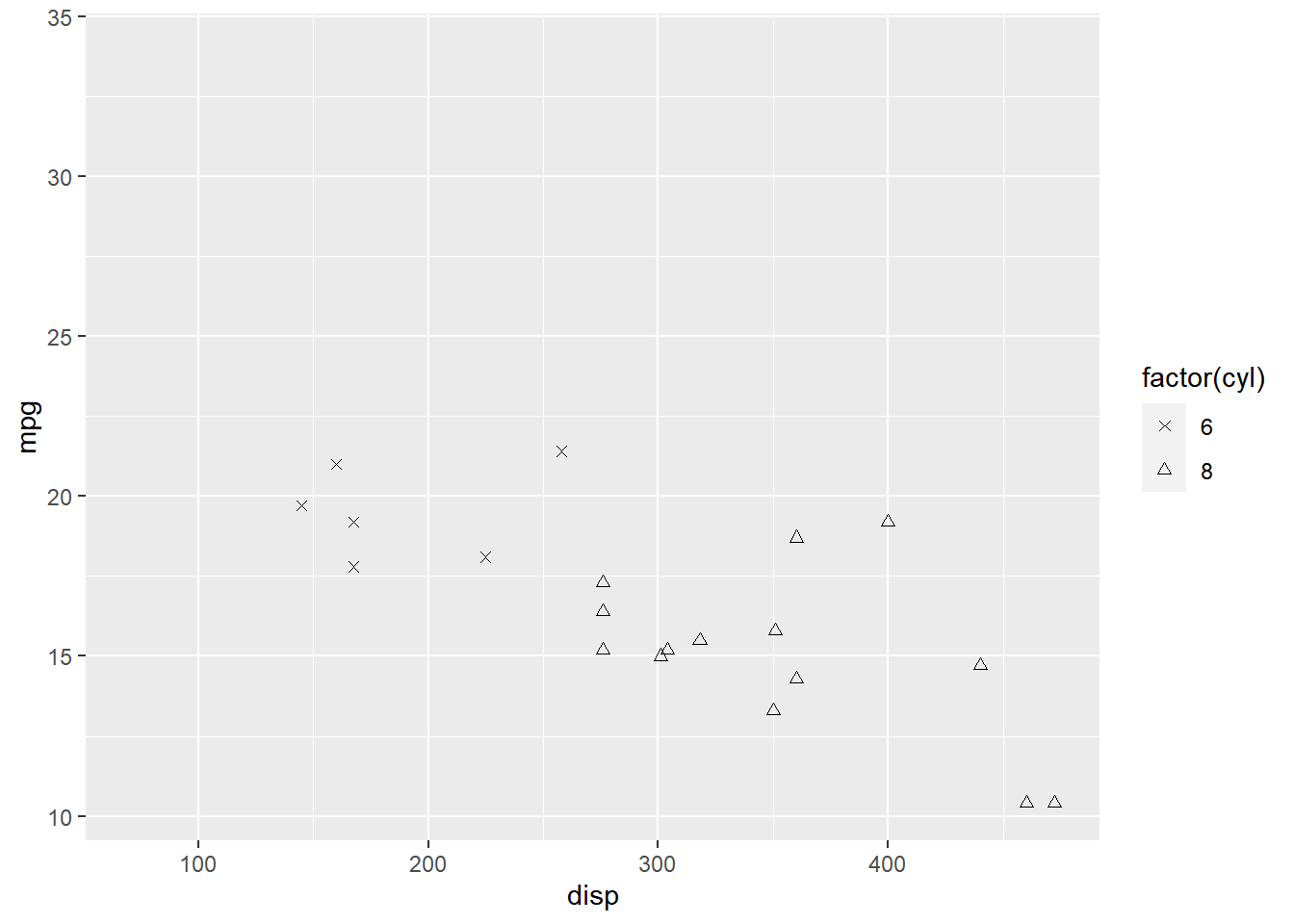ggplot2: Legend – Part 3

March 7, 2018
By

(This article was first published on Rsquared Academy Blog, and kindly contributed to R-bloggers)

Introduction

This is the 15th post in the series Elegant Data Visualization with ggplot2.
In the previous post, we learnt how to modify the legend of plots when
aesthetics are mapped to variables..In this post, we will learn to modify the
following using scale_shape_manual when shape is mapped to categorical
variables:

• title
• breaks
• limits
• labels
• values

Libraries, Code & Data

We will use the following libraries in this post:

All the data sets used in this post can be found here and code can be downloaded from here.

Plot

Let us start with a scatter plot examining the relationship between displacement
and miles per gallon from the mtcars data set. We will map the shape of the points
to the cyl variable.

ggplot(mtcars) +
geom_point(aes(disp, mpg, shape = factor(cyl)))As you can see, the legend acts as a guide for the shape aesthetic. Now, let
us learn to modify the different aspects of the legend.

Title

The title of the legend (factor(cyl)) is not very intuitive. If the user does
not know the underlying data, they will not be able to make any sense out of it.
Let us change it to Cylinders using the name argument.

ggplot(mtcars) +
geom_point(aes(disp, mpg, shape = factor(cyl))) +
scale_shape_manual(name = "Cylinders", values = c(4, 12, 24))If you have mapped shape/size to a discrete variable which has less than six
categories, you can use scale_shape().

ggplot(mtcars) +
geom_point(aes(disp, mpg, shape = factor(cyl))) +
scale_shape(name = 'Cylinders')Values

To change the default shapes in the legend, use the values argument and
supply a numeric vector of shapes. The number of shapes specified
must be equal to the number of levels in the categorical variable mapped.
In the below example, cyl has 3 levels (4, 6, 8) and hence we have specified
3 different shapes.

ggplot(mtcars) +
geom_point(aes(disp, mpg, shape = factor(cyl))) +
scale_shape_manual(values = c(4, 12, 24))Labels

The labels in the legend can be modified using the labels argument. Let us
change the labels to Four, Six and Eight in the next example. Ensure that
the labels are intuitive and easy to interpret for the end user of the plot.

ggplot(mtcars) +
geom_point(aes(disp, mpg, shape = factor(cyl))) +
scale_shape_manual(values = c(4, 12, 24), labels = c('Four', 'Six', 'Eight'))Limits

Let us assume that we want to modify the data to be displayed i.e. instead of
examining the relationship between mileage and displacement for all cars, we
desire to look at only cars with at least 6 cylinders. One way to approach this
would be to filter the data using filter from dplyr and then visualize it.
Instead, we will use the limits argument and filter the data for visualization.

ggplot(mtcars) +
geom_point(aes(disp, mpg, shape = factor(cyl))) +
scale_shape_manual(values = c(4, 24), limits = c(6, 8))
## Warning: Removed 11 rows containing missing values (geom_point).As you can see above, ggplot2 returns a warning message indicating data related
to 4 cylinders has been dropped. If you observe the legend, it now represents
only 4 and 6 cylinders.

Breaks

When there are large number of levels in the mapped variable, you may not
want the labels in the legend to represent all of them. In such cases, we can
use the breaks argument and specify the labels to be used. In the below case,
we use the breaks argument to ensure that the labels in legend represent
two levels (4, 8) of the mapped variable.

ggplot(mtcars) +
geom_point(aes(disp, mpg, shape = factor(cyl))) +
scale_shape_manual(values = c(4, 12, 24), breaks = c(4, 8))Putting it all together

ggplot(mtcars) +
geom_point(aes(disp, mpg, shape = factor(cyl))) +
scale_shape_manual(name = "Cylinders", labels = c('Six', 'Eight'),
values = c(4, 24), limits = c(6, 8), breaks = c(6, 8))
## Warning: Removed 11 rows containing missing values (geom_point).Summary

In this post, we learnt to modify the following aspects of legends:

• title
• breaks
• limits
• labels
• values

Up Next..

In the next post, we will learn how to modify the legend when size is mapped to variables.

R-bloggers.com offers daily e-mail updates about R news and tutorials on topics such as: Data science, Big Data, R jobs, visualization (ggplot2, Boxplots, maps, animation), programming (RStudio, Sweave, LaTeX, SQL, Eclipse, git, hadoop, Web Scraping) statistics (regression, PCA, time series, trading) and more...

If you got this far, why not subscribe for updates from the site? Choose your flavor: e-mail, twitter, RSS, or facebook...